# Java Swing | JComboBox with examples

JComboBox is a part of Java Swing package. JComboBox inherits JComponent class . JComboBox shows a popup menu that shows a list and the user can select a option from that specified list . JComboBox can be editable or read- only depending on the choice of the programmer .
Constructor of the JComboBox are:

1. JComboBox() : creates a new empty JComboBox .
2. JComboBox(ComboBoxModel M) : creates a new JComboBox with items from specified ComboBoxModel
3. JComboBox(E [ ] i) : creates a new JComboBox with items from specified array.
4. JComboBox(Vector items) : creates a new JComboBox with items from the specified vector

Commonly used Methods are :

3. getItemAt(int i) : returns the item at index i
4. getItemCount(): returns the number of items from the list
5. getSelectedItem() : returns the item which is selected
6. removeItemAt(int i) : removes the element at index i
7. setEditable(boolean b) : the boolean b determines whether the combo box is editable or not .If true is passed then the combo box is editable or vice versa.
8. setSelectedIndex(int i): selects the element of JComboBox at index i.
9. showPopup() :causes the combo box to display its popup window.
10. setUI(ComboBoxUI ui): sets the L&F object that renders this component.
11. setSelectedItem(Object a): sets the selected item in the combo box display area to the object in the argument.
12. setSelectedIndex(int a): selects the item at index anIndex.
13. setPopupVisible(boolean v): sets the visibility of the popup.
14. setModel(ComboBoxModel a) : sets the data model that the JComboBox uses to obtain the list of items.
15. setMaximumRowCount(int count): sets the maximum number of rows the JComboBox displays.
16. setEnabled(boolean b): enables the combo box so that items can be selected.
17. removeItem(Object anObject) : removes an item from the item list.
18. removeAllItems(): removes all items from the item list.
19. removeActionListener(ActionListener l): removes an ActionListener.
20. isPopupVisible() : determines the visibility of the popup.
22. getActionCommand() : returns the action command that is included in the event sent to action listeners.
23. getEditor(): returns the editor used to paint and edit the selected item in the JComboBox field.
24. getItemCount() : returns the number of items in the list.
25. getItemListeners(): returns an array of all the ItemListeners added to this JComboBox with addItemListener().
26. createDefaultKeySelectionManager() : returns an instance of the default key-selection manager.
27. fireItemStateChanged(ItemEvent e) : notifies all listeners that have registered interest for notification on this event type.
28. firePopupMenuCanceled() : notifies PopupMenuListeners that the popup portion of the combo box has been canceled.
29. firePopupMenuWillBecomeInvisible() : notifies PopupMenuListeners that the popup portion of the combo box has become invisible.
30. firePopupMenuWillBecomeVisible() : notifies PopupMenuListeners that the popup portion of the combo box will become visible.
31. setEditor(ComboBoxEditor a): sets the editor used to paint and edit the selected item in the JComboBox field.
32. setActionCommand(String a) : sets the action command that should be included in the event sent to actionListeners.
33. getUI() : returns the look and feel object that renders this component.
34. paramString() : returns a string representation of this JComboBox.
35. getUIClassID() : returns the name of the Look and feel class that renders this component.
36. getAccessibleContext() : gets the AccessibleContext associated with this JComboBox

The following programs will illustrate the use of JComboBox
1. Program to create a simple JComboBox and add elements to it .

## Java

 `// Java Program to create a simple JComboBox ` `// and add elements to it` `import` `java.awt.event.*;` `import` `java.awt.*;` `import` `javax.swing.*;` `class` `solve ``extends` `JFrame ``implements` `ItemListener {`   `    ``// frame` `    ``static` `JFrame f;`   `    ``// label` `    ``static` `JLabel l, l1;`   `    ``// combobox` `    ``static` `JComboBox c1;`   `    ``// main class` `    ``public` `static` `void` `main(String[] args)` `    ``{` `        ``// create a new frame` `        ``f = ``new` `JFrame(``"frame"``);`   `        ``// create a object` `        ``solve s = ``new` `solve();`   `        ``// set layout of frame` `        ``f.setLayout(``new` `FlowLayout());`   `        ``// array of string containing cities` `        ``String s1[] = { ``"Jalpaiguri"``, ``"Mumbai"``, ``"Noida"``, ``"Kolkata"``, ``"New Delhi"` `};`   `        ``// create checkbox` `        ``c1 = ``new` `JComboBox(s1);`   `        ``// add ItemListener` `        ``c1.addItemListener(s);`   `        ``// create labels` `        ``l = ``new` `JLabel(``"select your city "``);` `        ``l1 = ``new` `JLabel(``"Jalpaiguri selected"``);`   `        ``// set color of text` `        ``l.setForeground(Color.red);` `        ``l1.setForeground(Color.blue);`   `        ``// create a new panel` `        ``JPanel p = ``new` `JPanel();`   `        ``p.add(l);`   `        ``// add combobox to panel` `        ``p.add(c1);`   `        ``p.add(l1);`   `        ``// add panel to frame` `        ``f.add(p);`   `        ``// set the size of frame` `        ``f.setSize(``400``, ``300``);`   `        ``f.show();` `    ``}` `    ``public` `void` `itemStateChanged(ItemEvent e)` `    ``{` `        ``// if the state combobox is changed` `        ``if` `(e.getSource() == c1) {`   `            ``l1.setText(c1.getSelectedItem() + ``" selected"``);` `        ``}` `    ``}` `}`

Output :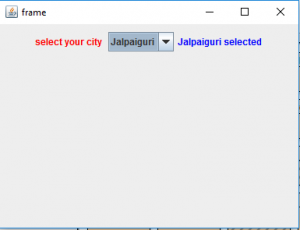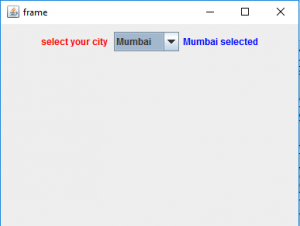2. Program to create two checkbox one editable and other read only

## Java

 `// Java Program to create two  checkbox ` `// one editable and other read only` `import` `java.awt.event.*;` `import` `java.awt.*;` `import` `javax.swing.*;` `class` `solve ``extends` `JFrame ``implements` `ItemListener {`   `    ``// frame` `    ``static` `JFrame f;`   `    ``// label` `    ``static` `JLabel l, l1, l3, l4;`   `    ``// combobox` `    ``static` `JComboBox c1, c2;`   `    ``// main class` `    ``public` `static` `void` `main(String[] args)` `    ``{` `        ``// create a new frame` `        ``f = ``new` `JFrame(``"frame"``);`   `        ``// create a object` `        ``solve s = ``new` `solve();`   `        ``// array of string containing cities` `        ``String s1[] = { ``"Jalpaiguri"``, ``"Mumbai"``, ``"Noida"``, ``"Kolkata"``, ``"New Delhi"` `};` `        ``String s2[] = { ``"male"``, ``"female"``, ``"others"` `};`   `        ``// create checkbox` `        ``c1 = ``new` `JComboBox(s1);` `        ``c2 = ``new` `JComboBox(s2);`   `        ``// set Kolkata and male as selected items` `        ``// using setSelectedIndex` `        ``c1.setSelectedIndex(``3``);` `        ``c2.setSelectedIndex(``0``);`   `        ``// add ItemListener` `        ``c1.addItemListener(s);` `        ``c2.addItemListener(s);`   `        ``// set the checkbox as editable` `        ``c1.setEditable(``true``);`   `        ``// create labels` `        ``l = ``new` `JLabel(``"select your city "``);` `        ``l1 = ``new` `JLabel(``"Jalpaiguri selected"``);` `        ``l3 = ``new` `JLabel(``"select your gender "``);` `        ``l4 = ``new` `JLabel(``"Male selected"``);`   `        ``// set color of text` `        ``l.setForeground(Color.red);` `        ``l1.setForeground(Color.blue);` `        ``l3.setForeground(Color.red);` `        ``l4.setForeground(Color.blue);`   `        ``// create a new panel` `        ``JPanel p = ``new` `JPanel();`   `        ``p.add(l);`   `        ``// add combobox to panel` `        ``p.add(c1);`   `        ``p.add(l1);`   `        ``p.add(l3);`   `        ``// add combobox to panel` `        ``p.add(c2);`   `        ``p.add(l4);`   `        ``// set a layout for panel` `        ``p.setLayout(``new` `FlowLayout());`   `        ``// add panel to frame` `        ``f.add(p);`   `        ``// set the size of frame` `        ``f.setSize(``400``, ``400``);`   `        ``f.show();` `    ``}` `    ``public` `void` `itemStateChanged(ItemEvent e)` `    ``{` `        ``// if the state combobox 1is changed` `        ``if` `(e.getSource() == c1) {`   `            ``l1.setText(c1.getSelectedItem() + ``" selected"``);` `        ``}`   `        ``// if state of combobox 2 is changed` `        ``else` `            ``l4.setText(c2.getSelectedItem() + ``" selected"``);` `    ``}` `}`

Output :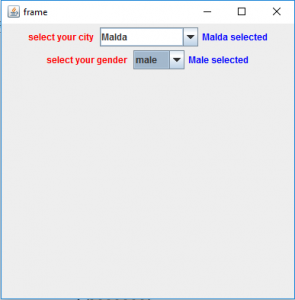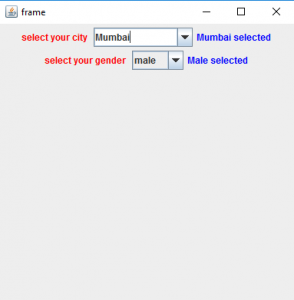3. Program to create a checkbox and add or remove items from it .

## Java

 `// Java  Program to create a checkbox ` `// and add or remove items from it` `import` `java.awt.event.*;` `import` `java.awt.*;` `import` `javax.swing.*;` `class` `solve11 ``extends` `JFrame ``implements` `ItemListener, ActionListener {`   `    ``// frame` `    ``static` `JFrame f;`   `    ``// label` `    ``static` `JLabel l, l1;`   `    ``// combobox` `    ``static` `JComboBox c1;`   `    ``// textfield to add and delete items` `    ``static` `JTextField tf;`   `    ``// main class` `    ``public` `static` `void` `main(String[] args)` `    ``{` `        ``// create a new frame` `        ``f = ``new` `JFrame(``"frame"``);`   `        ``// create a object` `        ``solve11 s = ``new` `solve11();`   `        ``// set layout of frame` `        ``f.setLayout(``new` `FlowLayout());`   `        ``// array of string containing cities` `        ``String s1[] = { ``"Jalpaiguri"``, ``"Mumbai"``, ``"Noida"``, ``"Kolkata"``, ``"New Delhi"` `};`   `        ``// create checkbox` `        ``c1 = ``new` `JComboBox(s1);`   `        ``// create textfield` `        ``tf = ``new` `JTextField(``16``);`   `        ``// create add and remove buttons` `        ``JButton b = ``new` `JButton(``"ADD"``);` `        ``JButton b1 = ``new` `JButton(``"REMOVE"``);`   `        ``// add action listener` `        ``b.addActionListener(s);` `        ``b1.addActionListener(s);`   `        ``// add ItemListener` `        ``c1.addItemListener(s);`   `        ``// create labels` `        ``l = ``new` `JLabel(``"select your city "``);` `        ``l1 = ``new` `JLabel(``"Jalpaiguri selected"``);`   `        ``// set color of text` `        ``l.setForeground(Color.red);` `        ``l1.setForeground(Color.blue);`   `        ``// create a new panel` `        ``JPanel p = ``new` `JPanel();`   `        ``p.add(l);`   `        ``// add combobox to panel` `        ``p.add(c1);`   `        ``p.add(l1);` `        ``p.add(tf);` `        ``p.add(b);` `        ``p.add(b1);`   `        ``f.setLayout(``new` `FlowLayout());`   `        ``// add panel to frame` `        ``f.add(p);`   `        ``// set the size of frame` `        ``f.setSize(``700``, ``200``);`   `        ``f.show();` `    ``}` `    ``// if button is pressed` `    ``public` `void` `actionPerformed(ActionEvent e)` `    ``{` `        ``String s = e.getActionCommand();` `        ``if` `(s.equals(``"ADD"``)) {` `            ``c1.addItem(tf.getText());` `        ``}` `        ``else` `{` `            ``c1.removeItem(tf.getText());` `        ``}` `    ``}`   `    ``public` `void` `itemStateChanged(ItemEvent e)` `    ``{` `        ``// if the state combobox is changed` `        ``if` `(e.getSource() == c1) {`   `            ``l1.setText(c1.getSelectedItem() + ``" selected"``);` `        ``}` `    ``}` `}`

Output :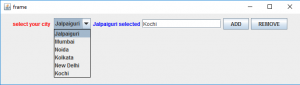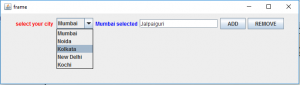Note : the above programs might not run in an online compiler please use an offline IDE

Whether you're preparing for your first job interview or aiming to upskill in this ever-evolving tech landscape, GeeksforGeeks Courses are your key to success. We provide top-quality content at affordable prices, all geared towards accelerating your growth in a time-bound manner. Join the millions we've already empowered, and we're here to do the same for you. Don't miss out - check it out now!

Previous
Next